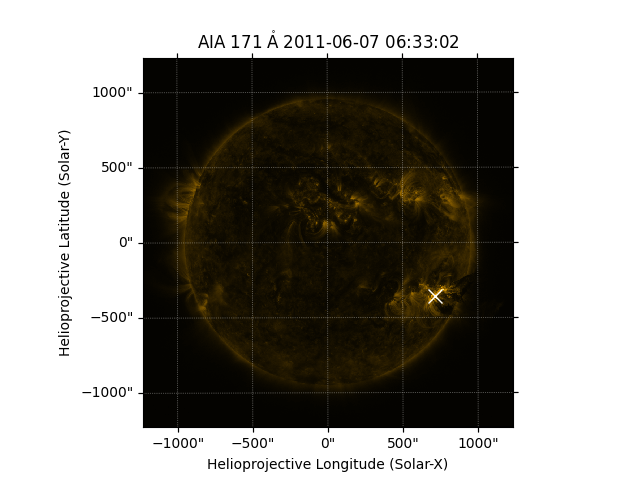Finding the brightest pixel¶

How to find and overplot the location of the brightest pixel.

import matplotlib.pyplot as plt
import numpy as np

import astropy.units as u

import sunpy.map
from sunpy.data.sample import AIA_171_IMAGE

aia = sunpy.map.Map(AIA_171_IMAGE)

To find the brightest pixel, we find the maximum in the AIA image data then transform that pixel coordinate to a map coordinate.

pixel_pos = np.argwhere(aia.data == aia.data.max()) * u.pixel
hpc_max = aia.pixel_to_world(pixel_pos[:, 1], pixel_pos[:, 0])

Let’s now plot the results.

fig = plt.figure()
ax = plt.subplot(projection=aia)
aia.plot()
ax.plot_coord(hpc_max, 'wx', fillstyle='none', markersize=10)
plt.show()Total running time of the script: ( 0 minutes 0.422 seconds)

Gallery generated by Sphinx-Gallery